### IMO Shortlist 2013 problem A3

Kvaliteta:
Avg: 4,0
Težina:
Avg: 7,0
Let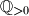$\mathbb{Q}_{>0}$ be the set of positive rational numbers. Let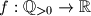$f : \mathbb{Q}_{>0} \to \mathbb{R}$ be a function satisfying the conditions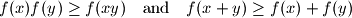for all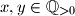$x, y \in \mathbb{Q}_{>0}$. Given that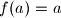$f(a) = a$ for some rational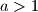$a > 1$, prove that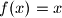$f(x) = x$ for all$x \in \mathbb{Q}_{>0}$.
Izvor: Bulgaria